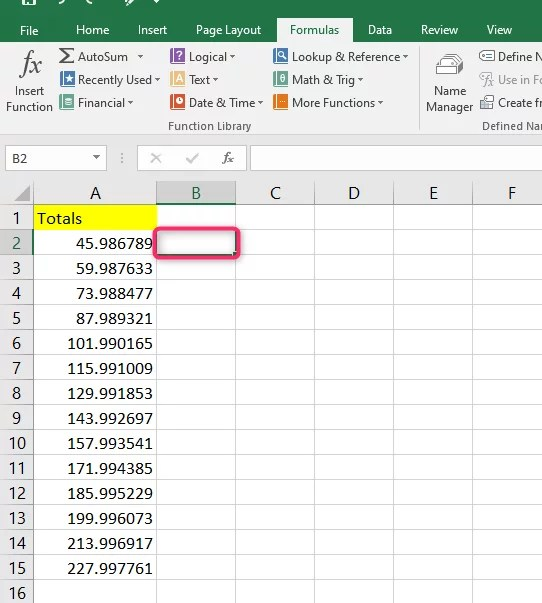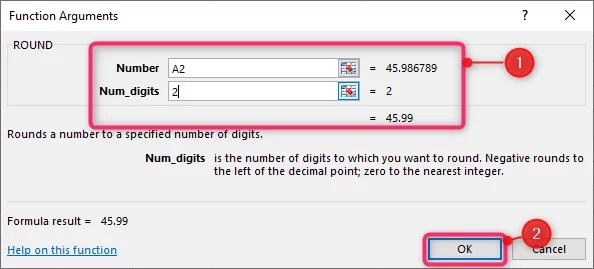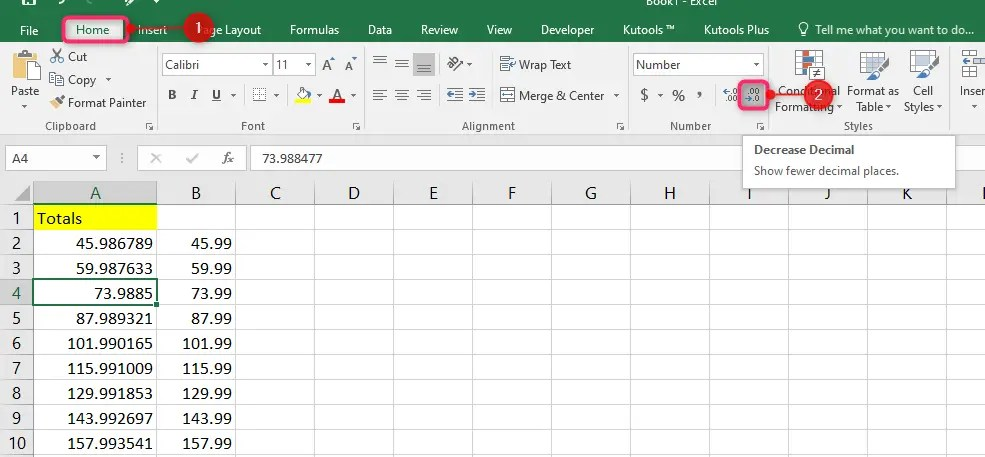# How to round off in Excel

It is common to come across decimal values when you are working with Excel data. But you can simplify your numerical data by using the ROUND functions. Excel offers its users three ROUND functions, namely: ROUNDUP, ROUND, and ROUNDDOWN. All these functions serve different purposes. Here we discuss how you can round off in Excel using the ROUND functions. Let’s get started.

## Benefits of Rounding Off numbers in Excel

Rounding off numbers can have several benefits, including:

Simplicity: Rounded numbers are often easier to work with and understand than long, complex decimals. For example, rounding \$1.5678\$ to \$1.57\$ makes the number easier to work with in calculations.

Clarity: Rounding off can make it easier to communicate numbers to others. It’s often easier to communicate a rounded number such as “about 500” rather than an exact number like “482.736”.

Estimation: Rounding off numbers can be useful for estimation purposes. For example, if you’re trying to calculate a rough estimate of the cost of a new car, rounding off the price to the nearest thousand dollars can give you a quick estimate without having to do a detailed calculation.

Precision: Rounding off can help to avoid errors in calculations. If you’re dealing with very large or small numbers with many decimal places, rounding off can help you avoid mistakes due to rounding errors.

Consistency: Rounding off can help to maintain consistency when working with a set of numbers. For example, if you’re dealing with a set of measurements, rounding all of them to the same number of decimal places can make it easier to compare and analyze the data.

Overall, rounding off can make calculations and communication simpler, faster, and more accurate.

## Using the ROUND function to round off in Excel

When you use the ROUND function, Excel will round off your numerical data to the number of decimal places you configure. When rounding off, if the next digit right after the decimal point (to the right) is between 0 and 4, it will automatically round down. But if your digit is higher than four, it will round up. For instance, 104.3245 will be 104.32 or 104, while 104.3254 will be 104.33 or 105.

1. In an open Excel workbook, select the cell where you want your rounded results to be displayed.2. Click on the Formulas tab on the main menu ribbon.

3. In the Function Library group, click on the option Math & Trig to display a drop-down list.

4. On the Math & Trig drop-down list, click on the option ROUND to select the function. The action will open up a dialog window.5. In the Functions Arguments dialog window, on the Number field, type in or select the number you want to round. You can type manually or access your worksheet and reference a cell.

6. On the Num_Digits field, specify how many digits your resulting number should have. You can either select zero, a positive number, or a negative number. 0 (zero) will round to the nearest integer, a negative number (-1…-8) will round to the left of the decimal place, while a positive number (1….9) specifies the number of digits after the decimal points that will appear after rounding off.

7. Click the OK button.8. To apply this formula to the other cell, use the fill handle to drag the formula and apply it to the other cells.## Rounding off numbers to the nearest unit by using Excel commands

You can round off your data to the nearest major unit by using a function in a formula.

1. In an open Excel worksheet, select the cells that you want to format.

2. Go to the Home tab of the main menu ribbon.

3. Click the Increase Decimal or Decrease Decimal icon to determine how many digits you want to display after the decimal point.## Using number format to round off in Excel

1. In an open Excel worksheet, click on the Home tab of the main ribbon.

2. Go to the Number group and click the arrow next to the list of number formats.

3. Click on the options More Number Formats.

4. Under the Category list, click on either Currency, Percentage, Accounting, or Scientific. It will depend on the type of data you have.

5. Enter the number of decimal places you want in the Decimal Places box.## Using ROUNDUP and ROUNDDOWN functions

1. In an open Excel worksheet, click on the cell where you want to display your results.

2. Click on the Formulas tab of the main menu ribbon.

3. Click on Math & Trig.

4. Choose either ROUNDUP or ROUNDDOWN from the drop-down list.5. In the Function Arguments dialog window, fill in the Number and Num_digits fields.

6. Click OK when you are done to apply your changes.